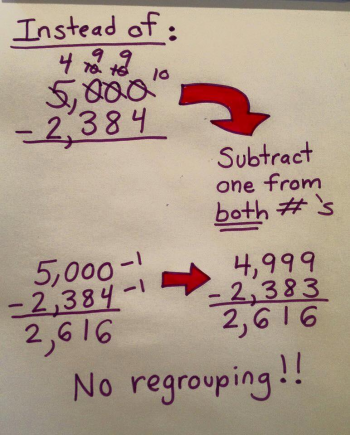Games
Problems
Go Pro!Erin shared the following image, and wants to know if this works. (Click on the image to see a larger version).

Well, Erin, there are really two questions here, and I'm going to try to answer both of them. The first question is the one you asked - Does it work? The second question is one you didn't ask - Is it practical?

## Does it Work?

When you ask if it works, I assume you really mean, "Does it work all the time? Or just for this specific example?"

The answer to that is, yes, it always works. I can show you some simple algebra to help you see that this always works.

Suppose you have the equation A - B = C (In this case, A = 5000, B = 2384, and C = 2616)

If we subtract 1 from the number we start with, and the number we're subtracting from, we get:

(A - 1) - (B - 1). If we distribute that negative in front of (B - 1), we get an equivalent expression: A - 1 - B + 1. But the -1 and the +1 are like terms which can be combined, to give A - B, which is still C. So yes, this always works.

What's more, an altered version of this process works with addition:

Suppose you wanted to add 9999 + 4352. Add one to 9999 to get 10000 and subtract one from 4352 to get 4351. Now do 10000 + 4351, and you have 14351.

## Is It Practical?

If you are trying to do the problem mentally, then yes, I would say this is practical. However, if you're trying to do it on paper, you should consider that it actually takes more writing to write the entire problem over again, instead of making the few marks required to indicate where you've borrowed. Of course, if you don't understand regrouping/borrowing, then the method shown here will be very appealing to you, even if it takes more writing.

I was trying to think of a real world application where this would be useful, and it didn't take much thought. Suppose I'm at the store, and I have a ten dollar bill. I want to buy an item that costs \$3.54. What will my change be?

Instead of mentally trying to do \$10.00 - \$3.54, I can mentally do \$9.99 - \$3.53 = \$6.46.Like us on Facebook to get updates about new resources